## Self-Consistent Renormalization Theory of Anisotropic Spin Fluctuations in Quaasi-One Dimensional Nearly Antiferromagnetic Metals

Rikio Konno1*

1Full Professor, Kindai University Group, Kindai University Technical College, 7-1 Kasugaoka, Nabari-shi, Mie 518-0459, Japan.

*Corresponding Author:Rikio Konno, Kindai University Technical College, 7-1 Kasugaoka, Nabari-shi, Mie 518-0459, Japan, Telephone: 81-595-41-0111; Fax: 81-595-62-1320; Email-id: r-konno@ktc.ac.jp

Citation: Rikio Konno (2023) Self-Consistent Renormalization Theory of Anisotropic Spin Fluctuations in Quaasi-One Dimensional Nearly Antiferromagnetic Metals. Nano Technol & Nano Sci J 5:135.

Received: February 14, 2023; Accepted: February 15, 2023; Published: February 24, 2023.

Copyright: © 2023 Rikio Konno, et al. This is an open-access article distributed under the terms of the Creative Commons Attribution License, which permits unrestricted use, distribution, and reproduction in any medium, provided the original author and source are credited.

## Abstract

Self-consistent renormalization theory of anisotropic spin fluctuations in quasi-one dimensional nearly antiferromagnetic metal was made. The inverse of the staggered magnetic susceptibility, the nuclear magnetic relaxation rate, the linear coefficient of the specific heat was investigated within this theory. The staggered magnetic susceptibility was composed of the longitudinal staggered magnetic susceptibility and the transverse staggered magnetic susceptibility. We find that the inverse of the staggered magnetic susceptibility has anisotropy. We also find that the both of the inverse of the staggered magnetic susceptibility has T2 -dependence at low temperatures and that they have T-linear dependence at elevated temperatures. The nuclear magnetic relaxation rate has anisotropy.

## Introduction

Many researchers have been interested in itinerant nearly antiferromagnetic metals.1–11) Moriya’s group developed the self-consistent renormalization theory of spin fluctuations (SCR theory).5–11) The SCR theory succeeded in explaining the physical properties of the nearly antiferromagnetic metals. However, the magnetic properties of the quasi-one dimensional nearly antiferromagnetic metals have not been resolved theoretically. The anisotropic spin fluctuations in quasi-one dimensional nearly antiferromagnetic metal is taken into account beyond the random phase approximation self-consistntly by SCR theory. This paper is organized as follows: the inverse of the staggered magnetic susceptibility will be provided in the next section. The nuclear magnetic relaxation rate will be supplied in section 3. The linear coefficient of the specific heat will be provided in section 4. The last section will give the conclusions. Throughout this paper, we use units of energy, such that ? = 1, kB = 1, and gµB = 1 where g is the g-factor of the conduction electron, unless explicitly stated.

The Inverse of The Staggered Magnetic Susceptibility with The SCR Theory in A Quasi-One-Dimensional Wave Number Space

By using Moriya’s expression12) based on the single band Hubbard model, the non-interacting dynamical susceptibility χ (Q + q, ω) in quasi-one-dimensional systems as follows:13)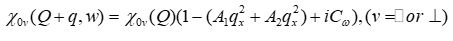(1)

where A2 << A1 in quasi-one dimension. the imaginary part of the dynamical susceptibility(2)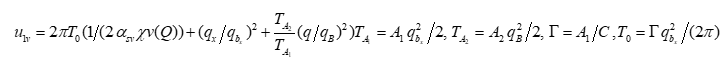(3)(4)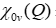is the non-interacting staggered magnetic susceptibility.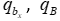are zone boundary wave lengths. The square of the local spin amplitude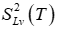is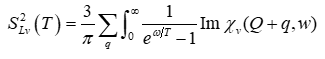(5)

From Eq. (2),is(6)

Where,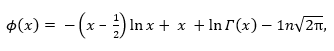(7)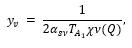(8)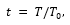(9)

By following Ref.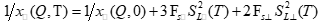(10)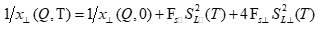(11)

The following inverse of the reduced staggered magnetic susceptibility is introduced.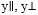are parallel to the a-axis and perpendicular to a-axis, respectively.(12)

Figure 1: The temperature dependence of the inverse of the reduced staggered magnetic susceptibility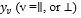when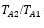=0.15 with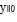= 0.02,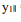= 1(the red line), y0 = 0.08, y1 = 3(the orange line), respectively.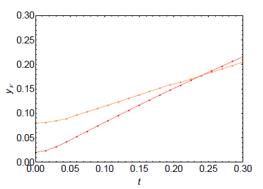Figure 2: The temperature dependence of the inverse of the reduced staggered magnetic susceptibilitywhen=0.3 with= 0.02,= 1(the red line), y0 = 0.08, y1 = 3(the orange line), respectively.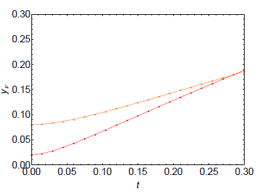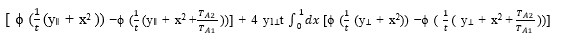(13)

where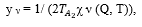(14)(15)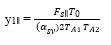(16)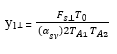(17)

Figure 3: The temperature dependence of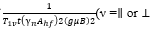when= 015, with y0=0.08(the orange line),=0.02(the red line), y=1,= 3, respectively.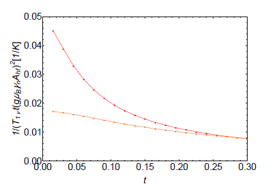Figs.1 and 2 show the temperature dependence of yν with various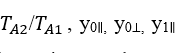and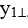The inverse of the reduced staggered magnetic susceptibility has T-linear dependence from Figs.1 and. 2. At low temperatures t<< 1, we use the following asymtotic expansion of the gamma function in the integrand of Eqs. (12) and (13).(18)

The inverse of the reduced staggered magnetic susceptibility yν is obtained by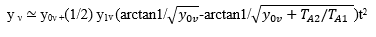(19)

The inverses of the staggered magnetic susceptibilities show T2-linear dependence at low temperatures. The inverse of the staggered magnetic susceptibility increases when spin fluctuations increase. It decreases whenincreases.

The Nuclear Magnetic Relaxation Rate

The nuclear magnetic relaxation rate is studied by using the dynamical susceptibility in the quasi-one dimensional nearly antiferromagnetic metals. It is obtained: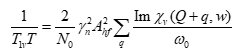(20)

where T1 is a nuclear spin relaxation time, Ah f is the hyperfine coupling constant. γn is the nuclear gyromagnetic ratio,and N0 is the number of the magnetic atom. The nuclear spin relaxation time in the quasi-one dimensional nearly antiferromagnetic metal is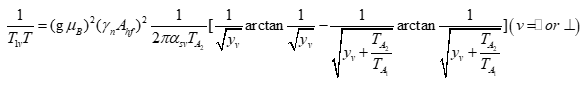(21)

where kB is the Boltzmann constant, g is the g-factor of the conduction electron, and μB is the Bohr’s magneton.

Figs. 3 and 4 show the temperature dependence of the nuclear magnetic relaxation rate. From Eq. (24),)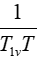has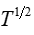linear dependence elevated temperatures.

Figure 4: The temperature dependence ofwhenTA2/TA1 = 0.3, with=0.08(the orange line),=0.02(the red line),=1,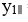= 3, respectively.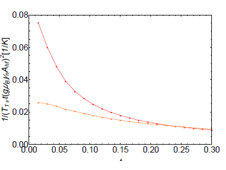The T-Linear Coefficient of The Specific Heat

The free energy of spin fluctuations is obtained as follows:5)(22)

with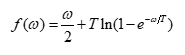(23)

where Γ is the damping constant of spin fluctuations. The specific heat of spin fluctuations is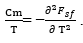( 24)

The T-linear coefficient of specific heat γm is obtained.(25)

Fig. 5 and Fig. 6 show the TA2/TA1dependence of the T-linear coefficient of the specific heat γm when T0=100K, T0=35K, respectively. From Fig. 5, γm decreases when TA2/TA1 increases. γm increases when y increases.

## Conclusions

We have studied the temperature dependence of the inverse of the staggered magnetic susceptibility by using anisotropic SCR theory in quasi-one dimensional nearly antiferromagnetic metal. The inverse of the staggered magnetic susceptibility has T2-linear dependence at low temperatures. With the increase of temperatures, it has T-linear dependence. The anisotropic staggered magnetic susceptibility is produced. The temperature dependence of the nuclear magnetic relaxation rate has been studied. The nuclear relaxation rate has T1/2- dependence.

Figure 5: TA2/TA1dependence of the T-linear coefficient of specific heat with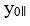= 0.02, y0 = 0.08, T0 = 100K (The black line). The orange line and the red line show the longitudinal contribution of the inverse of the magnetic staggered susceptibility and the transverse contribution of that, respectively.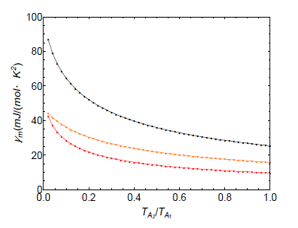Figure 6: TA2/TA1dependence of the T-linear coefficient of specific heat with= 0.02, y0 = 0.08, T0 = 35K, respectively.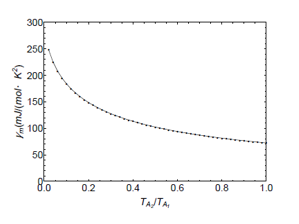## Acknowledgments

This work was supported by the Kindai University Technical College Grant. The author would like to thank Y. Takahashi and Y. Tokunaga for fruitful discussion. The author would like to also thank Y. Haga, H. von Lohneysen, M.Brando, F. Steglich, C. Geibel, J. Flouquet, A. de Visser, and S. Murayama for stimulating conversations.